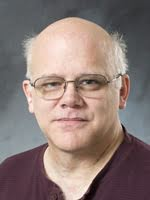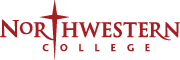Dr. Timothy Huffman Professor of Mathematics; Director of the Actuarial Science ProgramEducation:

712-707-7058
timh@nwciowa.edu
VPH 120

Dr. Huffman's research specialty is Fourier-Feynman Transforms. His work has been published in the International Journal of Mathematics and Mathematical Science, Transactions of the AMS, Rocky Mountain Journal of Mathematics, Michigan Mathematics Journal, and Journal of the Korean Mathematical Society. An academic correspondent of the Casualty Actuarial Society, he teaches many of the upper-level math classes at Northwestern.

Courses

Further topics in probability and statistics selected from: multiple regression, ANOVA, non-linear regression, covariance, collinearity, time series and auto-correlation.Prerequisite: C- or better in MAT116 or 117 or permission of instructor.(3 credits, alternate years, consult department)

MAT312 - Differential Equations

A study of the elementary theory, methods of solution, and applications of differential equations, which may include topics such as first order ordinary differential equations, linear equations with constant coefficients, series solutions, variation of parameters, Laplace Transforms, linear systems, partial differential equations, and Fourier Series.Prerequisite: C- or better in MAT211 or permission of department chair.(3 credits)

MAT316 - Probability and Statistics I

A post-calculus course in probability and statistics, which develops the theory of discrete and continuous distributions, expected value, random variables and inferential statistics. Prerequisites: C- or better in MAT116QR, 117QR, or 208QR and MAT112QR or permission of instructor. (3 credits; alternate years, consult department)

MAT416 - Probability and Statistics II

A continuation of Probability and Statistics I. Emphasis will be placed on functions of random variables and sampling distributions.Prerequisite: MAT316.(3 credits, alternate years, consult department)

Memberships

Mathematical Association of America

American Mathematical Society

Association of Christians in the Mathematical Sciences